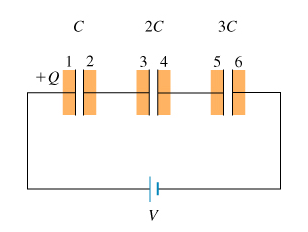# Problem: A.) What are the charges on plates 3 and 6?B.) If the voltage across the first capacitor (the one with capacitance C) is V', then what are the voltages across the second and third capacitors?C.) Find the voltage V1 across the first capacitor.D.) Find the charge Q on the first capacitor.E.) Using the value of Q just calculated, find the equivalent capacitance Ceq for this combination of capacitors in series.

###### FREE Expert Solution
86% (360 ratings)View Complete Written Solution
###### Problem DetailsA.) What are the charges on plates 3 and 6?

B.) If the voltage across the first capacitor (the one with capacitance C) is V', then what are the voltages across the second and third capacitors?

C.) Find the voltage V1 across the first capacitor.

D.) Find the charge Q on the first capacitor.

E.) Using the value of Q just calculated, find the equivalent capacitance Ceq for this combination of capacitors in series.